# 基于区域划分拓扑分析的GVF骨架线提取算法研究Research on GVF Skeletonization Extraction Algorithm Based on Topological Analysis of Regions Division

DOI: 10.12677/OE.2021.113015, PDF, HTML, XML, 下载: 44  浏览: 82  科研立项经费支持

Abstract: The purpose of this paper is to propose a new region division topology analysis algorithm to solve the problems of low precision and bifurcation in traditional skeleton extraction algorithm. When extracting the skeleton lines, the algorithm uses the method of region division, and extracts the topology in each region. Once the contradiction problem of breakpoint noise occurs, it divides again until the ideal skeleton line is obtained. Through the comparison, it is found that this new algorithm can get more complete and clear skeleton lines than the traditional algorithm, which provides a more powerful guarantee for the follow-up research.

1. 引言

2. 基于Snakes的GVF骨架线提取算法

Snakes算法 ，也称为主动轮廓算法，由Kass等人在1987年首次提出，该算法通过取定合适的初始值，能量函数最小化  来达到接近目标区域轮廓的目的。该算法的出现，使Snakes模型可以有效地应用于边缘检测和轮廓提取 ，从而正式让形变模型在图像分割上的应用一跃成为极具活力的研究领域。梯度矢量场最早是Xu和Prince等人以经典Snakes模型为基础，根据Helmholtz定理提出的，并建立了GVF Snakes  模型。在经典的可变形模型算法中只是包含静态场，而根据Helmholtz理论，可将一般的静态矢量场分解为一个同时包含无旋成分和无散成分的梯度矢量场，并设计了一个被称为梯度矢量力的外力，进而计算出图像的梯度场。

$-\alpha {v}_{ss}+\beta {v}_{ssss}+\nabla {E}_{ext}=0$ (1)

Xu和Prince等人，将经典Snakes模型的Euler方程看成是一个内外力的平衡方程，即：

${F}_{int}+{F}_{ext}=0$ (2)

$\epsilon =\iint \mu {|\nabla v|}^{2}+{|\nabla f|}^{2}{|v-\nabla f|}^{2}\text{d}x\text{d}y$ (3)

$\left\{\begin{array}{l}\mu {\nabla }^{2}v-\left(u-{f}_{x}\right)\left({f}_{x}^{2}+{f}_{y}^{2}\right)=0\\ \mu {\nabla }^{2}v-\left(u-{f}_{y}\right)\left({f}_{x}^{2}+{f}_{y}^{2}\right)=0\end{array}$ (4)

${\left(\frac{\partial \left(u,v\right)}{\partial \left(x,y\right)}\right)}_{\left({x}_{0},{y}_{0}\right)}={\left(\begin{array}{cc}\frac{\partial u}{\partial x}& \frac{\partial u}{\partial y}\\ \frac{\partial v}{\partial x}& \frac{\partial v}{\partial y}\end{array}\right)}_{\left({x}_{0},{y}_{0}\right)}$ (5)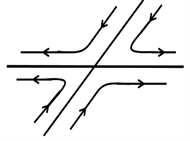(a) 鞍点μ < 0 < λ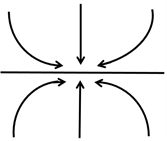(b) 结点μ < λ < 0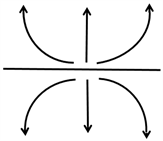(c) 排斥点0 < μ < λ

Figure 1. Critical point classification

3. 区域划分拓扑分析的GVF骨架线提取算法

3.1. 基于拓扑分析的GVF骨架线提取算法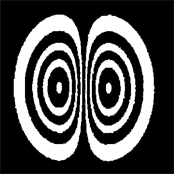(a) 圆环模型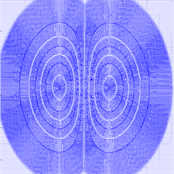(b) GVF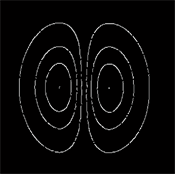(c) 骨架线

Figure 2. Original image, GVF and skeleton line of ring model(a) 原图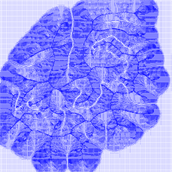(b) GVF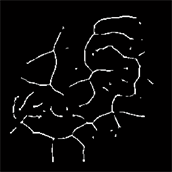(c) 骨架线(断点多)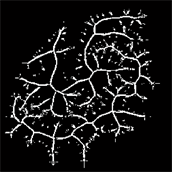(d) 骨架线(噪点多)

Figure 3. Original image, GVF and skeleton line of complex structure model

3.2. 区域划分拓扑分析的GVF骨架线提取算法(a) 俯视图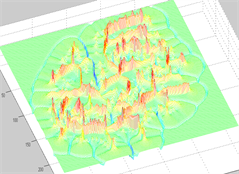(b) 立体图

Figure 4. Flux level map of complex structure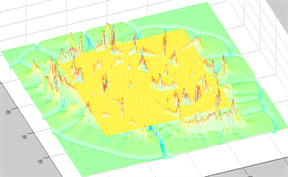(a) 原通量提取方式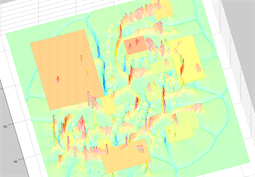(b) 现通量提取方式

Figure 5. Comparison of flux extraction methods for complex image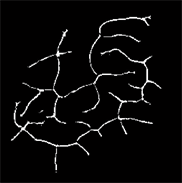Figure 6. Skeleton line extraction results based on region partition topology analysisTable 1. Comparison of two algorithms for skeleton extraction

4. 结论

NOTES

*通讯作者。

  Hisada, M., Belyaev, et al. (2002) A Skeleton-based Approach for Detection of Perceptually Salient Features on Polygonal Surfaces. Computer Graphics Forum, 21, 689-700. https://doi.org/10.1111/1467-8659.00627  Attali, D., Boissonnat, J.D. and Edelsbrunner, H. (2009) Stability and Computation of Medial Axes: A State-of-the-Art Report. In: Möller, T., Hamann, B. and Russell, R.D., Eds., Mathematical Foundations of Scientific Visualization, Computer Graphics, and Massive Data Exploration, Springer, Berlin, Heidelberg. https://doi.org/10.1007/b106657_6  Eftekharian, A.A. and Ilie, H.T. (2009) Distance Functions and Skeletal Representations of Rigid and Non-Rigid Planar Shapes. Computer Aided Design, 41, 865-876. https://doi.org/10.1016/j.cad.2009.05.006  Yan, H.W. (2010) Fundamental Theories of Spatial Similarity Relations in Multi-scale Map Spaces. Chinese Geographical Science, 20, 18-22. https://doi.org/10.1007/s11769-010-0018-z  Liu, X.F., Wu, Y.L. and Hu, H. (2013) A Method of Extracting Multiscale Skeletons for Polygonal Shapes. Acta Geodaetica et Cartographica Sinica, 42, 585-594.  Chang, Y.H., Kwon, S.H. and Choi, H.I. (2012) Medial Axis Transform of a Planar Domain with Infinite Curvature Boundary Points. Computer Aided Geometric Design, 29, 281-295. https://doi.org/10.1016/j.cagd.2012.04.001  Smogavec, G. and Zalik, B. (2012) A Fast Algorithm for Constructing Approximate Medial Axis of Polygons, Using Steiner Points. Advances in Engineering Software, 52, 1-9. https://doi.org/10.1016/j.advengsoft.2012.05.006  Zhou, Y. and Toga, A.W. (1999) Efficient Skeletonization of Volumetric Objects. IEEE Transactions on Visualization & Computer Graphics, 5, 196-209. https://doi.org/10.1109/2945.795212  Chung, D.H. and Sapiro, G. (2000) Segmentation-Free Skeletonization of Gray-Scale Images via PDEs. IEEE, 2, 927-930.  Xu, W., Chen, T., Zhang, J., et al. (2015) Two Parabolic-Hyperbolic Oriented Partial Differential Equations for Denoising in Electronic Speckle Pattern Interferometry Fringes. Applied Optics, 54, 4720-4726. https://doi.org/10.1364/AO.54.004720  Guo, B., Wang, T., Zhao, R., et al. (2010) Research on Algorithm of Polygon Skeleton Line Extracting. Bulletin of Surveying and Mapping, 33, 17-19.  Montero, A.S and Lang, J. (2012) Skeleton Pruning by Contour Approximation and the Integer Medial Axis Transform. Computers & Graphics, 36, 477-487. https://doi.org/10.1016/j.cag.2012.03.029  Ivanov, D., Kuzmin, E. and Burtsev, S. (2000) Efficient Integer-Based Skeletonization Algorithm. Computers & Graphics, 24, 41-51. https://doi.org/10.1016/S0097-8493(99)00136-3  Xu, C. and Prince, J.L. (1998) Snakes, Shapes, and Gradient Vector Flow. IEEE Transactions on Image Processing, 7, 359-369. https://doi.org/10.1109/83.661186  Helman, J. and Hesselink, L. (1989) Representation and Display of Vector Field Topology in Fluid Flow Data Sets. Computer, 22, 27-36. https://doi.org/10.1109/2.35197  Kass, M., Witkin, A., Terzopoulos. (1988) Snakes: Active Contour Models. International Journal of Computer Vision, 1, 321-331. https://doi.org/10.1007/BF00133570  Qin, L., Zhu, C., Zhao, Y., et al. (2013) Generalized Gradient Vector Flow for Snakes: New Observations, Analysis, and Im-provement. IEEE Transactions on Circuits & Systems for Video Technology, 23, 883-897. https://doi.org/10.1109/TCSVT.2013.2242554  Cohen, L. and Cohen, I. (1999) Finite Element Methods for Active Contour Models and Balloons for 2D and 3D Images. IEEE Transactions on Pattern Analysis and Machine Intelligence, 15, 1131-1147. https://doi.org/10.1109/34.244675  Peikert, R. and Sadlo, F. (2007) Topology-Guided Visualization of Constrained Vector Fields. In: Hauser, H., Hagen, H and Theisel, H., Eds., Topology-Based Methods in Visualization, Springer, Berlin, Heidelberg, 21-33. https://doi.org/10.1007/978-3-540-70823-0_2  Helman, J.L. and Hesselink, L. (1991) Visualizing Vector Field Topology in Fluid Flows. IEEE Computer Graphics and Applications, 11, 36-46. https://doi.org/10.1109/38.79452